pburg

Autoregressive power spectral density estimate — Burg’s method

Description

pxx = pburg(x,order) returns the power spectral density (PSD) estimate, pxx, of a discrete-time signal, x, found using Burg’s method. When x is a vector, it is treated as a single channel. When x is a matrix, the PSD is computed independently for each column and stored in the corresponding column of pxx. pxx is the distribution of power per unit frequency. The frequency is expressed in units of rad/sample. order is the order of the autoregressive (AR) model used to produce the PSD estimate.

example

pxx = pburg(x,order,nfft) uses nfft points in the discrete Fourier transform (DFT). For real x, pxx has length (nfft/2+1) if nfft is even, and (nfft+1)/2 if nfft is odd. For complex–valued x, pxx always has length nfft. If you omit nfft, or specify it as empty, then pburg uses a default DFT length of 256.

[pxx,w] = pburg(___) returns the vector of normalized angular frequencies, w, at which the PSD is estimated. w has units of rad/sample. For real-valued signals, w spans the interval [0,π] when nfft is even and [0,π) when nfft is odd. For complex-valued signals, w always spans the interval [0,2π).

example

[pxx,f] = pburg(___,fs) returns a frequency vector, f, in cycles per unit time. The sampling frequency, fs, is the number of samples per unit time. If the unit of time is seconds, then f is in cycles/second (Hz). For real-valued signals, f spans the interval [0,fs/2] when nfft is even and [0,fs/2) when nfft is odd. For complex-valued signals, f spans the interval [0,fs).

[pxx,w] = pburg(x,order,w) returns the two-sided AR PSD estimates at the normalized frequencies specified in the vector, w. The vector w must contain at least two elements, because otherwise the function interprets it as nfft.

[pxx,f] = pburg(x,order,f,fs) returns the two-sided AR PSD estimates at the frequencies specified in the vector, f. The vector f must contain at least two elements, because otherwise the function interprets it as nfft. The frequencies in f are in cycles per unit time. The sampling frequency, fs, is the number of samples per unit time. If the unit of time is seconds, then f is in cycles/second (Hz).

[___] = pburg(x,order,___,freqrange) returns the AR PSD estimate over the frequency range specified by freqrange. Valid options for freqrange are: 'onesided', 'twosided', or 'centered'.

[___,pxxc] = pburg(___,'ConfidenceLevel',probability) returns the probability × 100% confidence intervals for the PSD estimate in pxxc.

example

pburg(___) with no output arguments plots the AR PSD estimate in dB per unit frequency in the current figure window.

Examples

collapse all

Create a realization of an AR(4) wide-sense stationary random process. Estimate the PSD using Burg's method. Compare the PSD estimate based on a single realization to the true PSD of the random process.

Create an AR(4) system function. Obtain the frequency response and plot the PSD of the system.

A = [1 -2.7607 3.8106 -2.6535 0.9238];
[H,F] = freqz(1,A,[],1);
plot(F,20*log10(abs(H)))

xlabel('Frequency (Hz)')
ylabel('PSD (dB/Hz)')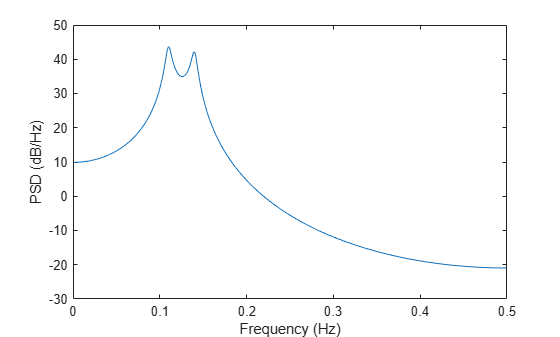Create a realization of the AR(4) random process. Set the random number generator to the default settings for reproducible results. The realization is 1000 samples in length. Assume a sampling frequency of 1 Hz. Use pburg to estimate the PSD for a 4th-order process. Compare the PSD estimate with the true PSD.

rng default

x = randn(1000,1);
y = filter(1,A,x);
[Pxx,F] = pburg(y,4,1024,1);

hold on
plot(F,10*log10(Pxx))
legend('True Power Spectral Density','pburg PSD Estimate')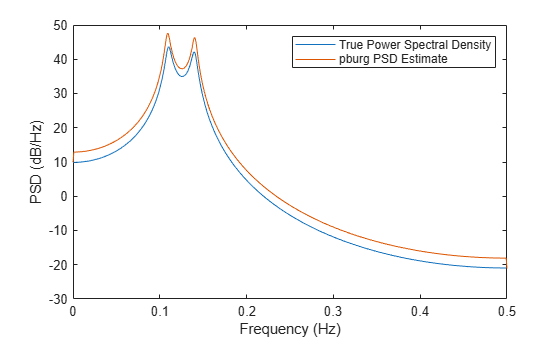Create a realization of an AR(4) process. Use arburg to determine the reflection coefficients. Use the reflection coefficients to determine an appropriate AR model order for the process. Obtain an estimate of the process PSD.

Create a realization of an AR(4) process 1000 samples in length. Use arburg with the order set to 12 to return the reflection coefficients. Plot the reflection coefficients to determine an appropriate model order.

A = [1 -2.7607 3.8106 -2.6535 0.9238];

rng default

x = filter(1,A,randn(1000,1));

[a,e,k] = arburg(x,12);

stem(k,'filled')
title('Reflection Coefficients')
xlabel('Model Order')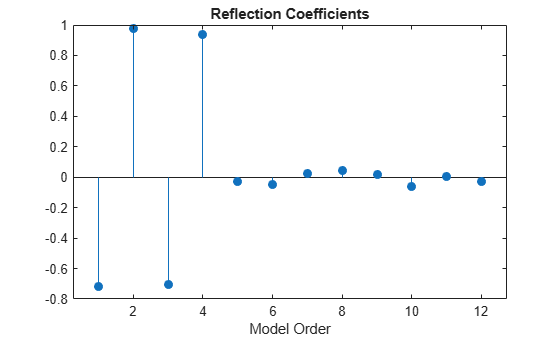The reflection coefficients decay to zero after order 4. This indicates an AR(4) model is most appropriate.

Obtain a PSD estimate of the random process using Burg's method. Use 1000 points in the DFT. Plot the PSD estimate.

pburg(x,4,length(x))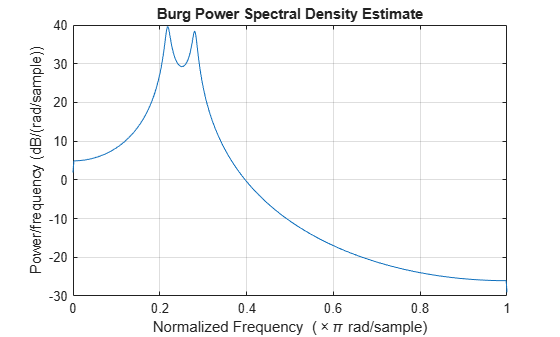Create a multichannel signal consisting of three sinusoids in additive $N\left(0,1\right)$ white Gaussian noise. The sinusoids' frequencies are 100 Hz, 200 Hz, and 300 Hz. The sampling frequency is 1 kHz, and the signal has a duration of 1 s.

Fs = 1000;

t = 0:1/Fs:1-1/Fs;

f = [100;200;300];

x = cos(2*pi*f*t)'+randn(length(t),3);

Estimate the PSD of the signal using Burg's method with a 12th-order autoregressive model. Use the default DFT length. Plot the estimate.

morder = 12;

pburg(x,morder,[],Fs)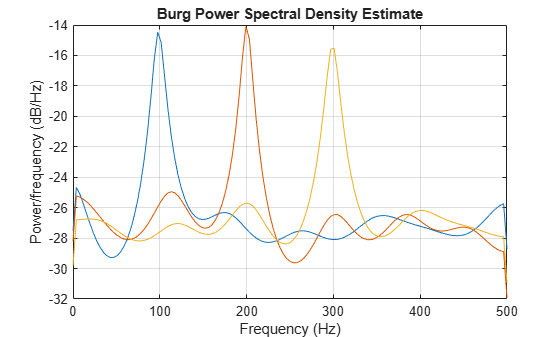Input Arguments

collapse all

Input signal, specified as a row or column vector, or as a matrix. If x is a matrix, then its columns are treated as independent channels.

Example: cos(pi/4*(0:159))+randn(1,160) is a single-channel row-vector signal.

Example: cos(pi./[4;2]*(0:159))'+randn(160,2) is a two-channel signal.

Data Types: single | double
Complex Number Support: Yes

Order of the autoregressive model, specified as a positive integer.

Data Types: double

Number of DFT points, specified as a positive integer. For a real-valued input signal, x, the PSD estimate, pxx has length (nfft/2+1) if nfft is even, and (nfft+1)/2 if nfft is odd. For a complex-valued input signal,x, the PSD estimate always has length nfft. If nfft is specified as empty, the default nfft is used.

Data Types: single | double

Sample rate, specified as a positive scalar. The sample rate is the number of samples per unit time. If the unit of time is seconds, then the sample rate has units of Hz.

Normalized frequencies, specified as a row or column vector with at least two elements. Normalized frequencies are in rad/sample.

Example: w = [pi/4 pi/2]

Data Types: double

Frequencies, specified as a row or column vector with at least two elements. The frequencies are in cycles per unit time. The unit time is specified by the sample rate, fs. If fs has units of samples/second, then f has units of Hz.

Example: fs = 1000; f = [100 200]

Data Types: double

Frequency range for the PSD estimate, specified as a one of 'onesided', 'twosided', or 'centered'. The default is 'onesided' for real-valued signals and 'twosided' for complex-valued signals. The frequency ranges corresponding to each option are

• 'onesided' — returns the one-sided PSD estimate of a real-valued input signal, x. If nfft is even, pxx has length nfft/2 + 1 and is computed over the interval [0,π] rad/sample. If nfft is odd, the length of pxx is (nfft + 1)/2 and the interval is [0,π) rad/sample. When fs is optionally specified, the corresponding intervals are [0,fs/2] cycles/unit time and [0,fs/2) cycles/unit time for even and odd length nfft respectively.

• 'twosided' — returns the two-sided PSD estimate for either the real-valued or complex-valued input, x. In this case, pxx has length nfft and is computed over the interval [0,2π) rad/sample. When fs is optionally specified, the interval is [0,fs) cycles/unit time.

• 'centered' — returns the centered two-sided PSD estimate for either the real-valued or complex-valued input, x. In this case, pxx has length nfft and is computed over the interval (–π,π] rad/sample for even length nfft and (–π,π) rad/sample for odd length nfft. When fs is optionally specified, the corresponding intervals are (–fs/2, fs/2] cycles/unit time and (–fs/2, fs/2) cycles/unit time for even and odd length nfft respectively.

Coverage probability for the true PSD, specified as a scalar in the range (0,1). The output, pxxc, contains the lower and upper bounds of the probability × 100% interval estimate for the true PSD.

Output Arguments

collapse all

PSD estimate, returned as a real-valued, nonnegative column vector or matrix. Each column of pxx is the PSD estimate of the corresponding column of x. The units of the PSD estimate are in squared magnitude units of the time series data per unit frequency. For example, if the input data is in volts, the PSD estimate is in units of squared volts per unit frequency. For a time series in volts, if you assume a resistance of 1 Ω and specify the sample rate in hertz, the PSD estimate is in watts per hertz.

Data Types: single | double

Normalized frequencies, returned as a real-valued column vector. If pxx is a one-sided PSD estimate, w spans the interval [0,π] if nfft is even and [0,π) if nfft is odd. If pxx is a two-sided PSD estimate, w spans the interval [0,2π). For a DC-centered PSD estimate, w spans the interval (–π,π] for even nfft and (–π,π) for odd nfft.

Data Types: double

Cyclical frequencies, returned as a real-valued column vector. For a one-sided PSD estimate, f spans the interval [0,fs/2] when nfft is even and [0,fs/2) when nfft is odd. For a two-sided PSD estimate, f spans the interval [0,fs). For a DC-centered PSD estimate, f spans the interval (–fs/2, fs/2] cycles/unit time for even length nfft and (–fs/2, fs/2) cycles/unit time for odd length nfft.

Data Types: double | single

Confidence bounds, returned as a matrix with real-valued elements. The row size of the matrix is equal to the length of the PSD estimate, pxx. pxxc has twice as many columns as pxx. Odd-numbered columns contain the lower bounds of the confidence intervals, and even-numbered columns contain the upper bounds. Thus, pxxc(m,2*n-1) is the lower confidence bound and pxxc(m,2*n) is the upper confidence bound corresponding to the estimate pxx(m,n). The coverage probability of the confidence intervals is determined by the value of the probability input.

Data Types: single | double